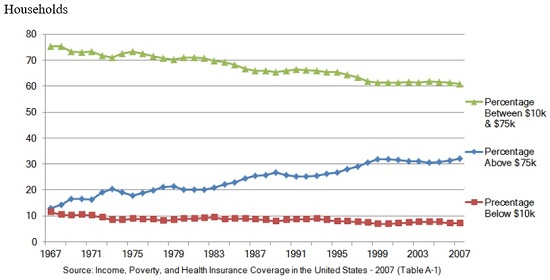# VCE Further Mathematics: Exam Prep & Study Guide Final Exam

Free Practice Test Instructions:

Choose your answer to the question and click 'Continue' to see how you did. Then click 'Next Question' to answer the next question. When you have completed the free practice test, click 'View Results' to see your results. Good luck!

#### Question 6 6. Given the following table of data of sales vs. time, what might the graph of the time plot of sales vs time with its 5-mean smoothed time plot superimposed look like?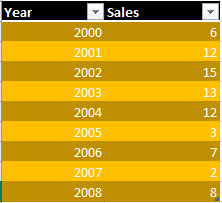#### Question 8 8. If this stem-and-leaf display is based on the data set below, then what is TRUE about the stem-and-leaf display? 10, 12, 7, 1, 4, 13, 7, 6, 13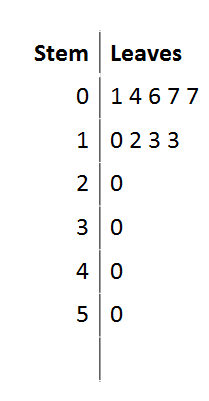#### Question 11 11. If the inverse of matrix A is this, what is the solution when the constants are 10 and 13?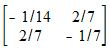#### Question 12 12. Use Cramer's rule to find the z solution to this linear system.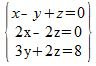#### Question 13 13. What is the y-intercept of this line graph?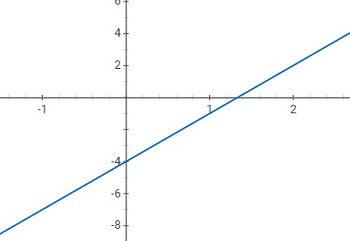#### Question 14 14. Which statement is true regarding the graph shown?